# De Moivre's formula

There are two distinct complex numbers z such that z3 is equal to 1 and z is not equal 1.

Calculate the sum of these two numbers.

Correct result:

S =  -1

#### Solution:

$z^3 = 1 \ \\ 1 = 1(\cos 0 + i \sin 0 ) \ \\ z_k = \sqrt{1}( \cos( \dfrac{2\pi k }{3} ) + i \sin( \dfrac{2\pi k }{3} ) ) \ \\ z_1 = 1 \ \\ z_2 = \cos( 120 ^\circ ) + i \sin( 120 ^\circ ) = -0.5 + 0.86602540378444 i \ \\ z_3 = \cos( 240 ^\circ ) + i \sin( 240 ^\circ ) = -0.5 - 0.86602540378444 i \ \\ S = z_2 + z_3 = -1$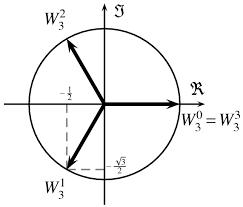Our examples were largely sent or created by pupils and students themselves. Therefore, we would be pleased if you could send us any errors you found, spelling mistakes, or rephasing the example. Thank you!

Leave us a comment of this math problem and its solution (i.e. if it is still somewhat unclear...):Be the first to comment!Tips to related online calculators

## Next similar math problems:

• Moivre 2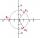Find the cube roots of 125(cos 288° + i sin 288°).
• Goniometric form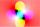Determine goniometric form of a complex number ?.
• Linear imaginary equationGiven that ? "this is z star" Find the value of the complex number z.
• The modulus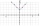Find the modulus of the complex number 2 + 5i
• Complex number coordinatesWhich coordinates show the location of -2+3i
• BearingA plane flew 50 km on a bearing 63°20' and the flew on a bearing 153°20' for 140km. Find the distance between the starting point and the ending point.A domed stadium is in the shape of spherical segment with a base radius of 150 m. The dome must contain a volume of 3500000 m³. Determine the height of the dome at its centre to the nearest tenth of a meter.Are these numbers 2i, 4i, 2i + 1, 8i, 2i + 3, 4 + 7i, 8i, 8i + 4, 5i, 6i, 3i complex?Find two imaginary numbers whose sum is a real number. How are the two imaginary numbers related? What is its sum?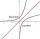Calculate value of expression log |3 +7i +5i2| .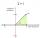Is -10i a positive number?Calculate reciprocal of z=0.8-1.8i:Calculate the absolute value of complex number -15-29i.